Jarvis' Blog (总有美丽的风景让人流连) 总有美丽的风景让人流连

# 智能剪刀 (Intelligent Scissors)

2020-07-09
Jarvis
Post

## 1. 概念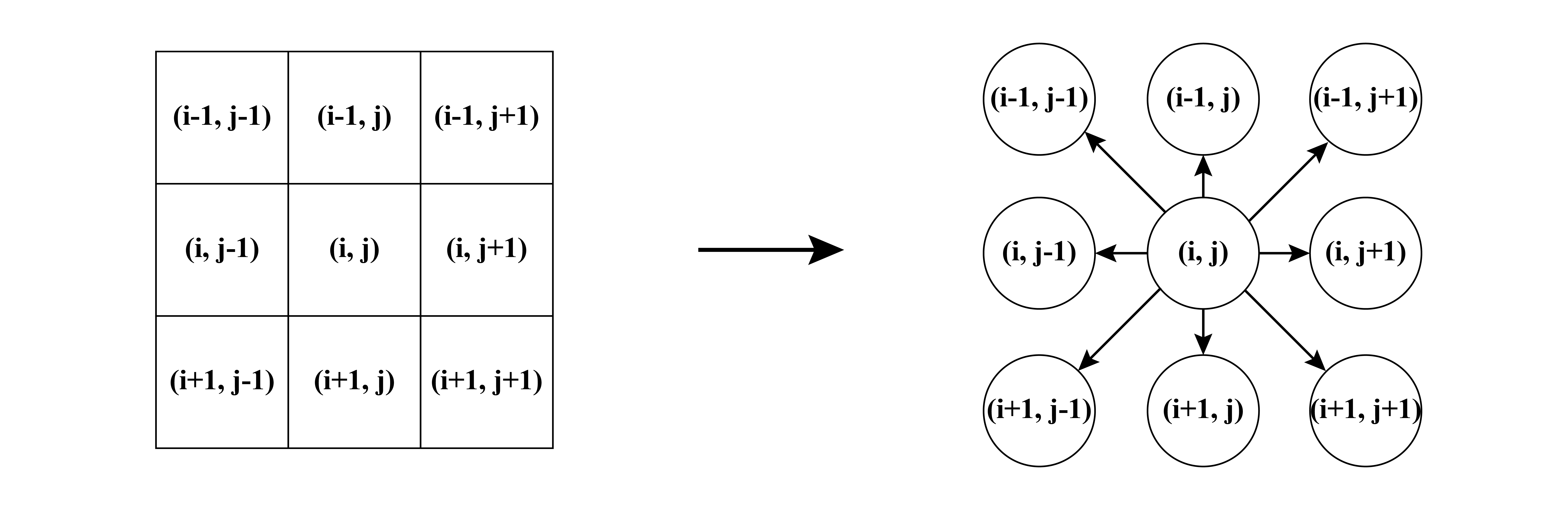1. 根据图像构造图, 包括节点和边, 使用八联通.
2. 为边计算损失, 包括静态权重和动态权重.
3. 用户在待分割目标的边界给定初始种子点
4. 即时计算上一种子点到鼠标点间的最短路径, 移动鼠标直到用户认为最短路径恰好就是图像的边界, 点击鼠标生成下一个种子点
5. 重复第4步直到目标物体的轮廓勾画完成.

## 2. 损失计算

Laplacian zero-crossing $$f_Z(\mathbf{q})$$ $$w_Z$$
Gradient magnitude $$f_G(\mathbf{q})$$ $$w_G$$
Gradient direction $$f_D(\mathbf{p},\mathbf{q})$$ $$w_D$$
Edge pixel value $$f_P(\mathbf{q})$$ $$w_P$$
“Inside” pixel value $$f_I(\mathbf{q})$$ $$w_I$$
“Outside” pixel value $$f_O(\mathbf{q})$$ $$w_O$$
• 前三种为静态损失, 得到图像后可以提前计算
• 后三种为动态损失, 在交互过程中实时计算

$\begin{multline*} l(\mathbf{p},\mathbf{q}) = M\cdot(\omega_Z\cdot f_Z(\mathbf{q}) + \omega_G\cdot f_G(\mathbf{q}) + \omega_D\cdot f_D(\mathbf{p}, \mathbf{q}) \\ +\omega_P\cdot f_P(\mathbf{q}) + \omega_I\cdot f_I(\mathbf{q}) + \omega_O\cdot f_O(\mathbf{q})) \end{multline*}$

### 2.1 Lapacian zero-crossing 拉普拉斯交叉零点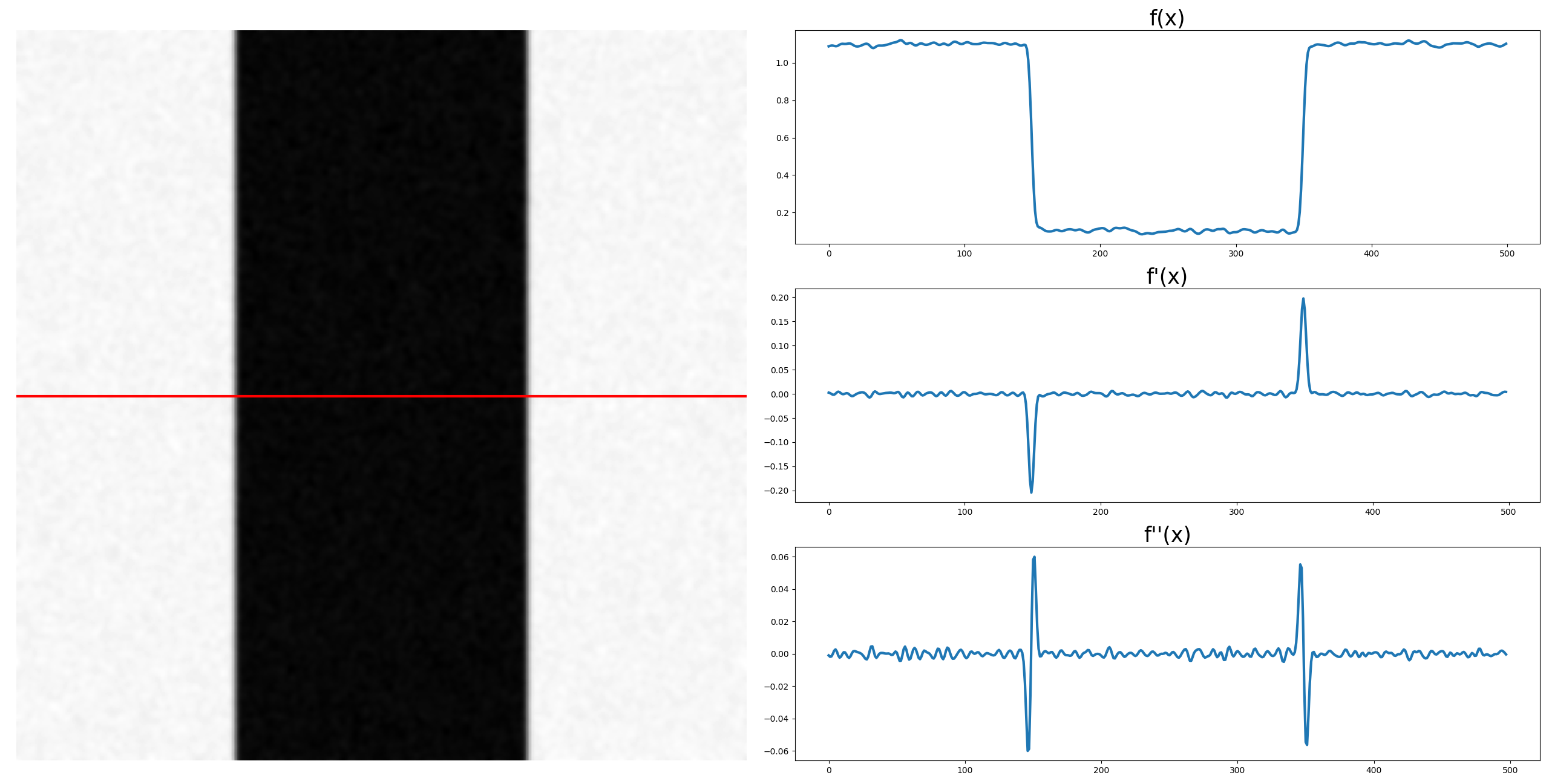\begin{align} G(x, y) &= \frac1{2\pi\sigma^2} \exp\left(-\frac{x^2+y^2}{2\sigma^2}\right),\\ \\ LoG(x, y) &= \Delta G(x, y) = \nabla^2G(x, y) \\ \\ &=- \frac1{\pi\sigma^4}(1-\frac{x^2+y^2}{2\sigma^2}) \exp\left(-\frac{x^2+y^2}{2\sigma^2}\right) \end{align}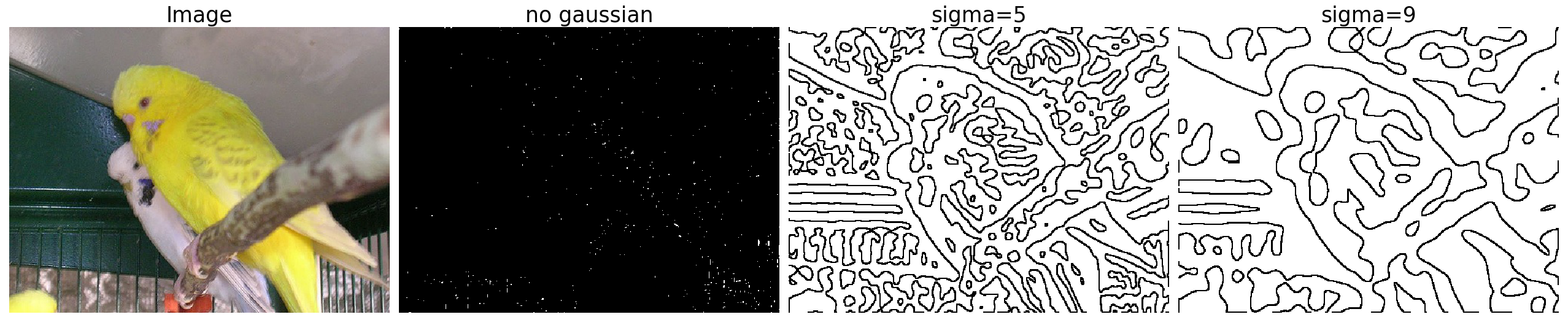$f_Z(\mathbf{q})=\begin{cases} 0;\quad \text{if}~I_L(\mathbf{q})=0, \\ 1;\quad \text{otherwise.} \end{cases}$

1. (某开源代码的方式) 判断点 q 的八个邻接点的 LoG, 如果这八个值中既有正数, 也有负数, 那么我们认为 q 是一个零交叉点, 把 $$f_Z(\mathbf{q})$$ 的值设为0, 其他为1. 这种方式的结果如上图所示.
2. (论文中的方式) 判断点 q 及其相邻的下一个点 (x 和 y 方向分别做), 如果两点的 LoG 异号, 那么选择两点中绝对值小的那个作为零交叉点. 这种方式的结果如下图所示.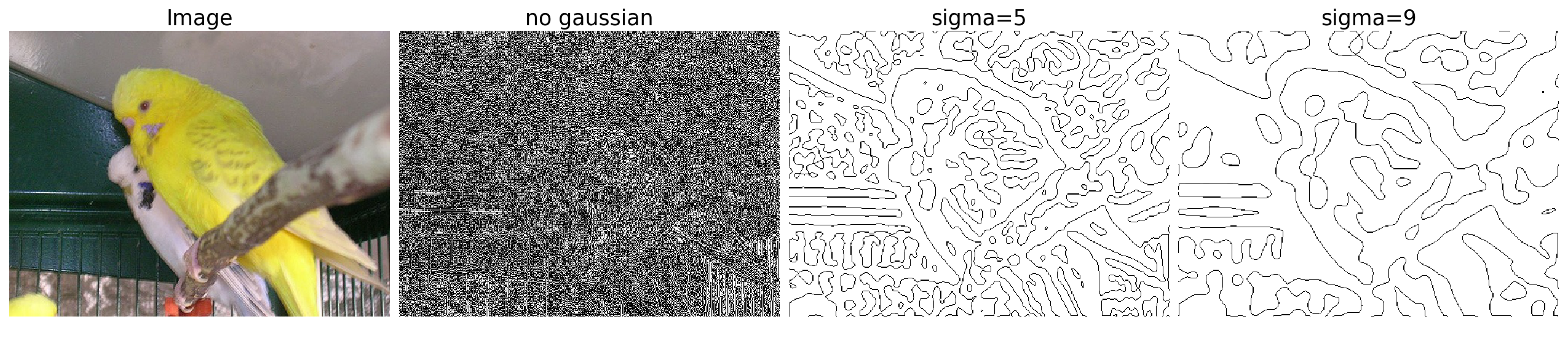• 拉普拉斯零交叉损失的尺寸: [h, w], 基于点 q 的.

$G=\sqrt{I_x^2+I_y^2}.$

$f_G = 1 - \frac{G - \min(G)}{\max(G) - \min(G)}$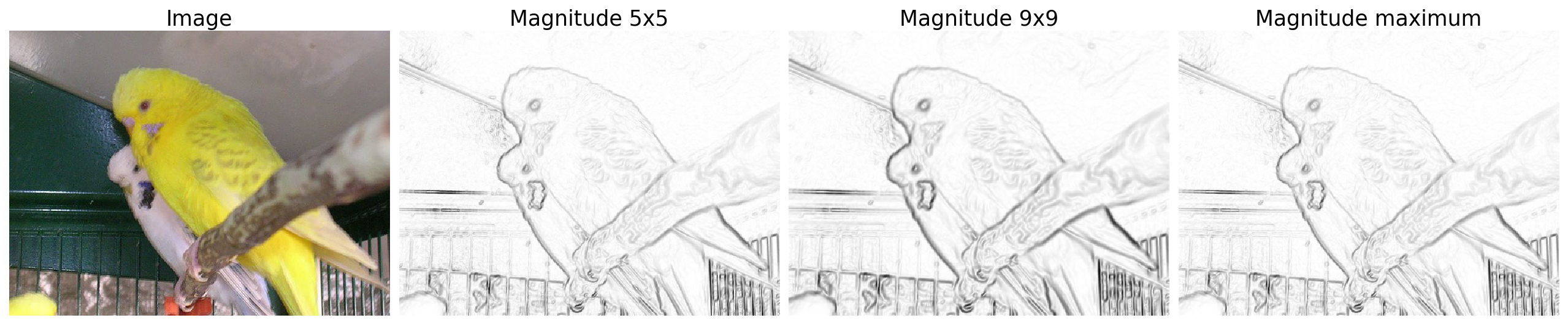1. 对多核的梯度大小使用 maximum, 取梯度大的核对应的梯度大小, 如上图所示.
2. 对多核的拉普拉斯零交叉点的斜率绝对值取 maximum. 取斜率绝对值大的核对应的梯度大小.

• 梯度大小损失的尺寸: [h, w], 基于点 q 的

$\mathbf{D(p)}=[I_x(\mathbf{p}), I_y(\mathbf{p})],\quad \mathbf{D'(p)}=[I_y(\mathbf{p}), -I_x(\mathbf{p})]$

$f_D(\mathbf{p},\mathbf{q}) = \frac2{3\pi} \left[ \arccos(d_\mathbf{p}(\mathbf{p},\mathbf{q})) + \arccos(d_\mathbf{q}(\mathbf{p},\mathbf{q}))\right]$

\begin{align} d_\mathbf{p}(\mathbf{p},\mathbf{q})) &= \mathbf{D'(p)\cdot L(p, q)} \\ d_\mathbf{q}(\mathbf{p},\mathbf{q})) &= \mathbf{L(p, q)\cdot D'(q)} \end{align}

$L(p,q)=\frac1{\Vert\mathbf{p-q}\Vert} \begin{cases} \mathbf{q-p};\quad \text{if}~~\mathbf{D'(p)\cdot(q-p)}\geq0 \\ \mathbf{p-q};\quad \text{otherwise} \end{cases}$

• 边的两个端点的梯度方向一致并且与边的方向也一致时损失较低
• 边的两个端点的梯度方向一致但与边的方向正交时损失较高
• 边的两个端点的梯度方向不一致时损失较高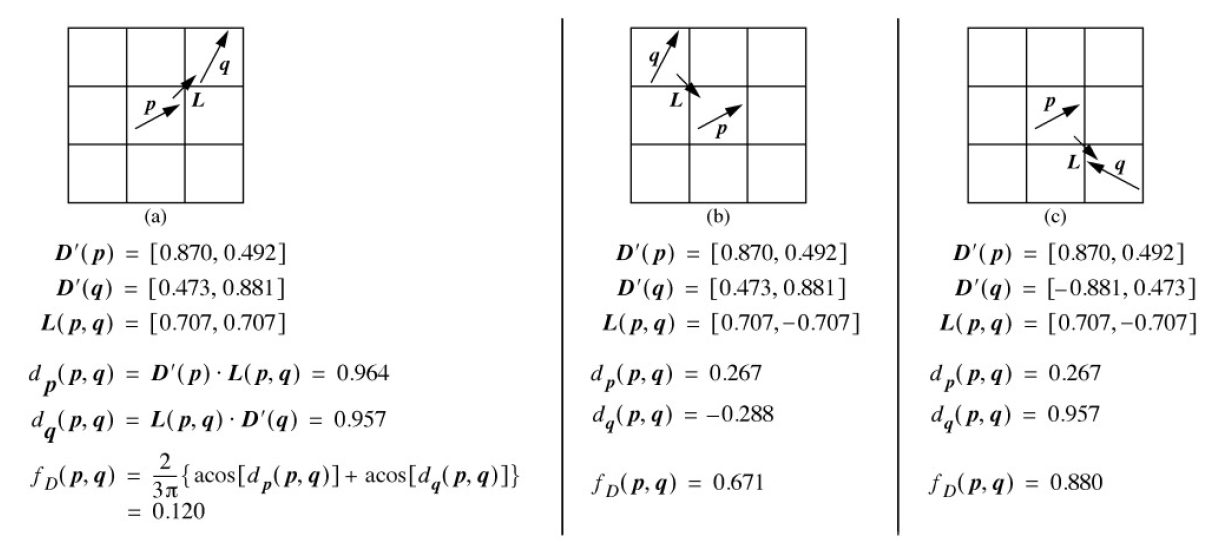• 梯度方向损失的尺寸: [3, 3, h, w], 基于边 p–q 的
• 以上三个损失中, 前两个损失需要统一做一个 unfold 的操作, 把 [h, w] 的尺寸变为(复制为) [3, 3, h, w], 最终得到一个四维张量 i,j,k,l, 其中 k 和 l 维度指代像素 p 的绝对坐标, 而 i 和 j 指代像素 p 的邻居 q 在 3x3 的小网格内的坐标.

### 2.4 Pixel value features 像素值特征

$f_P(\mathbf{p})=\frac1{255}I(\mathbf{p})$

$f_I(\mathbf{p})=\frac1{255}I(\mathbf{p}+k\cdot\mathbf{D(p)}) \\ f_O(\mathbf{p})=\frac1{255}I(\mathbf{p}-k\cdot\mathbf{D(p)})$

• 因为有些图像中, 梯度”强”的边未必是用户需要的边, 而”强”边附近的”弱”边可能是需要的. 在2.1-2.3中的三个静态损失下, 弱边是没有发言权的. 比如论文中的下图.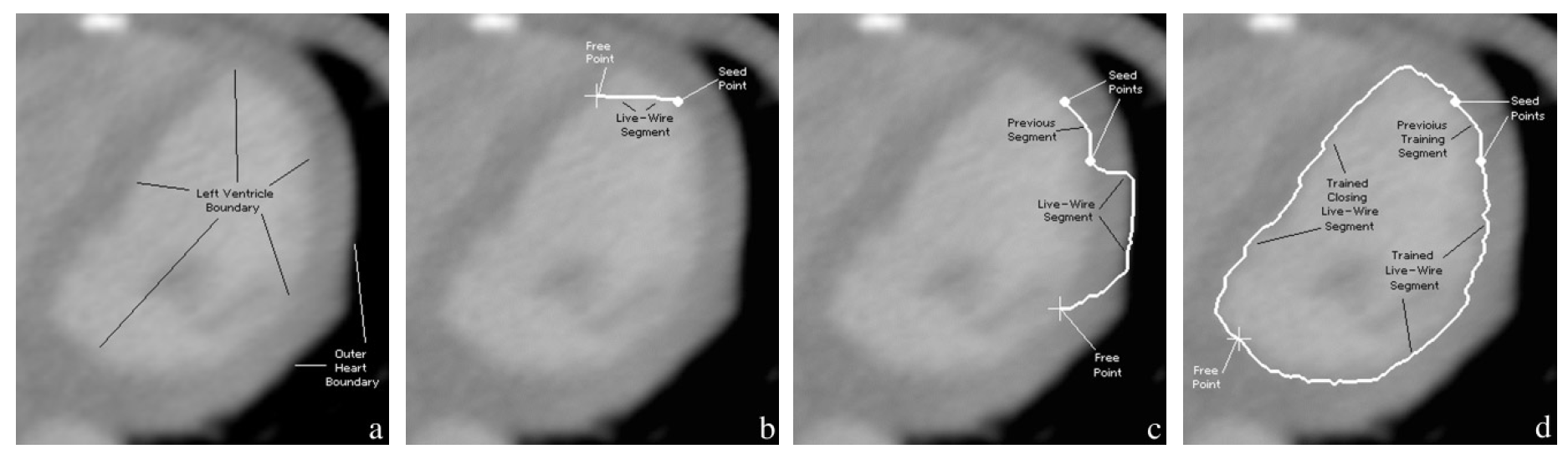$m_G=\left\lfloor M_G\left(1 - \frac{h_G}{\max(h_G)}\right)+0.5\right\rfloor$

$m_G'(x)=\begin{cases} \min\left(m_G(x), \left\lfloor M_G\left(1-\frac{x}{(n_G-1)}\left(1-\frac{t_s}{s}\right)\right)+0.5\right\rfloor\right); \quad &\text{if}~~t_s<s, \\ m_G(x); \quad &\text{otherwise}. \end{cases}$

1. 当用于训练的样本点的数量 $$t_s$$ 足够大时 (超过 s ), 那么我们认为 $$m_G$$ 作为梯度大小的直方图是可靠的, 因此可以用作梯度大小的损失函数.
2. 当没有训练样本的时候, 我们使用 2.2 节的 $$f_G$$ 作为梯度大小的损失.
3. 当 $$t_s$$ 不够大时 (小于 s ), 对于 $$m_G$$ 的信任就要打一个折扣, 那么实际的损失函数则使用情况1和情况2的组合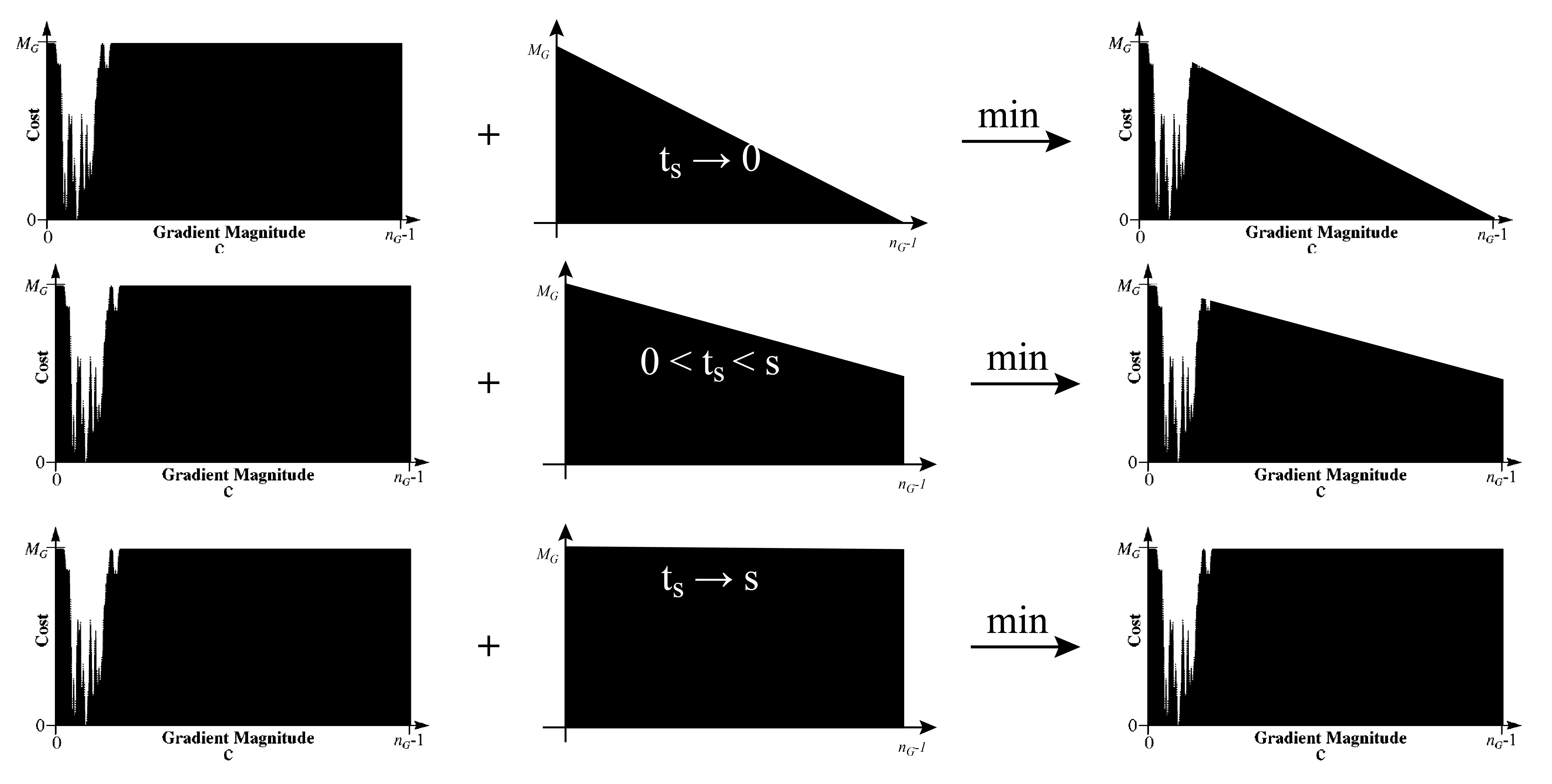$w_N(\mathbf{p}, \mathbf{q})=\begin{cases} 1; \quad &\mathbf{q}~\text{is a diagonal neighbor}, \\ 1/\sqrt{2};\quad &\mathbf{q}~\text{is a horizontal or vertical neighbor}. \end{cases}$

### 2.5 最终损失

$\begin{multline*} l'(\mathbf{p},\mathbf{q})=\lfloor M_Z\cdot f_Z(\mathbf{q})+0.5\rfloor + \lfloor M_D\cdot f_D(\mathbf{p},\mathbf{q})+0.5\rfloor \\ +w_N(\mathbf{p},\mathbf{q})\cdot m'_G(I_G(\mathbf{q})) +m_P(I_P(\mathbf{q})) +m_I(I_I(\mathbf{q})) +m_O(I_O(\mathbf{q})). \end{multline*}$

## 3. 实际操作和最短路径的计算

1. 计算两个静态损失, 和梯度大小的静态损失部分
2. 用户给定第一个种子点
3. 以种子点为中心, 提取图像的一个 NxN 的 patch, 在该 patch 上进行后续计算, 以减少计算量. (相当于 live wire 只在该种子点为中心边长为 N 的一个矩形区域内有效. 当然了图像不大或者算力足够时可以直接用全图计算.)
4. 在计算区域内使用 Dijkstra 单源最短路径算法计算所有像素点到刚点的种子点的最短路径
5. 根据鼠标位置实时更新当前的 live wire
6. 用户根据 live wire 确定一条最优路径, 点击鼠标生成下一个种子点, 则第一个种子点和下一个种子点之间的路径就完全确定.
7. 从前一步确定的路径末尾提取32/64个像素点作为训练集, 计算三个动态损失, 并更新图的权重 (注意要基于第1步的权重更新, 只对当前 live wire 有效. )
8. 返回第4步

## 4. 参考文献

1. Interactive segmentation with intelligent scissors
Mortensen E N, Barrett W A.
[html], [PDF]. In Graphical models and image processing, 1998, 60(5): 349-384.

2. Intelligent scissors for image composition
Mortensen E N, Barrett W A.
[html], [PDF]. In Proceedings of the 22nd annual conference on Computer graphics and interactive techniques. 1995: 191-198.

Content